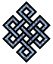#fail2ban bad ip database: ip 62.232.37.162

### | ip database | live view | stats | report | help | api key:

 ip: 62.232.37.162 hostname: 62.232.37.162 country:[GB] United Kingdom first reported: 16.11.2018 00:47.31 GMT+0200 last reported: 05.12.2018 06:02.24 GMT+0200 time period: 19d 05h 14m 53s total reports: 8 reported by: 3 host(s) filter(s): ssh (7) ssh (1) tor exit node no badips.com db Lookup## port scan of '62.232.37.162':

[-hide]
```# Nmap 6.40 scan initiated Fri Nov 16 00:48:02 2018 as: /usr/bin/nmap -sU -sS -O 62.232.37.162
Nmap scan report for 62.232.37.162
Host is up (0.020s latency).
Not shown: 999 open|filtered ports, 997 closed ports
PORT     STATE SERVICE
80/tcp   open  http
4443/tcp open  pharos
4444/tcp open  krb524
500/udp  open  isakmp
No exact OS matches for host (If you know what OS is running on it, see http://nmap.org/submit/ ).
TCP/IP fingerprint:
OS:SCAN(V=6.40%E=4%D=11/16%OT=80%CT=1%CU=%PV=N%G=Y%TM=5BEE05C8%P=x86_64-pc-
OS:linux-gnu)SEQ(SP=108%GCD=1%ISR=10A%TI=I%CI=Z%TS=U)OPS(O1=NNM590SNW0%O2=N
OS:NM578SNW0%O3=M280NW0%O4=NNM22CSNW0%O5=NNM218SNW0%O6=NNM109S)WIN(W1=FFFF%
OS:W2=FFFF%W3=FFFF%W4=FFFF%W5=FFFF%W6=FFFF)ECN(R=Y%DF=Y%TG=40%W=FFFF%O=NNM5
OS:90SNW0%CC=N%Q=)T1(R=Y%DF=Y%TG=40%S=O%A=S+%F=AS%RD=0%Q=)T2(R=N)T3(R=N)T4(
OS:R=Y%DF=Y%TG=40%W=0%S=A%A=Z%F=R%O=%RD=0%Q=)T5(R=Y%DF=Y%TG=40%W=0%S=Z%A=S+
OS:%F=AR%O=%RD=0%Q=)T6(R=Y%DF=Y%TG=40%W=0%S=A%A=Z%F=R%O=%RD=0%Q=)T7(R=N)U1(
OS:R=N)IE(R=N)

OS detection performed. Please report any incorrect results at http://nmap.org/submit/ .
# Nmap done at Fri Nov 16 00:48:24 2018 -- 1 IP address (1 host up) scanned in 22.94 seconds
```
```Σ = 34 | Δt = 0.0049519538879395s
```